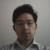三个数字相加小于某个值的所有可能组合这个算法怎么求，例如100,90,80，相加要少于500，可以有80+80，或者100，或者100+90+80

年薪达不到25W大数据工程师、拿不到Offer全额退款->>>1public class Q3376901_2262676 {

public static void main(String[] args) {
int[] num = new int[] { 100, 90, 80 };
int t = 500;
int[] count = new int[num.length];
s(num, count, 0, t);
}

public static void s(int[] num, int[] count, int i, int t) {
if (t <= 0 || i >= num.length) {
print(num, count);
return;
}

if (num[i] < t) {
count[i]++;
s(num, count, i, t - num[i]);
count[i]--;
}
s(num, count, i + 1, t);
}

private static void print(int[] num, int[] count) {
boolean f = true;
for (int i = 0; i < num.length; i++) {
for (int j = 0; j < count[i]; j++) {
if (!f) {
System.out.print("+");
} else {
f = false;
}
System.out.print(num[i]);
}
}
if (!f) {
System.out.println();
}
}
}